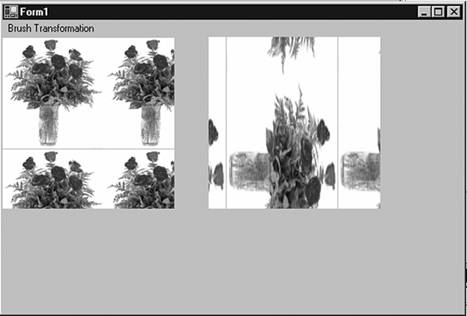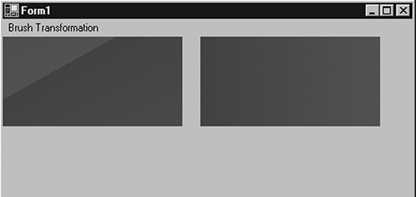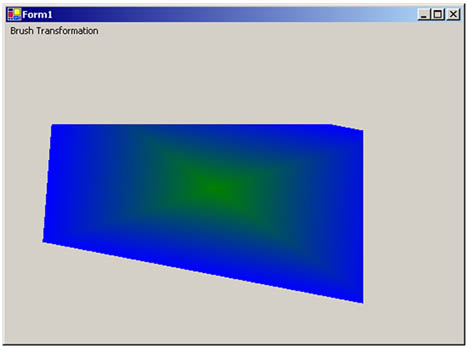# Transformation with Brushes

The TextureBrush, LinearGradientBrush, and PathGradientBrush classes also provide transformation methods. Brush transformation is not used very often, but it may be useful in some cases, as the following example will show.

A transformation on a TextureBrush object is a transformation of the image used as the texture. TextureBrush provides the methods MultiplyTransform, ResetTransform, RotateTransform, ScaleTransform, and TranslateTransform (see Table 4.15).

The TextureBrush class also provides a Transform property, which can be used to apply a transformation on a texture brush.

Table 4.15. TextureBrush methods

Method

Description

MultiplyTransform

Multiplies the Matrix object that represents the local geometric transformation of a texture brush by the specified Matrix object in the specified order.

ResetTransform

Resets the Transform property of a texture to identity.

RotateTransform

Rotates the local geometric transformation of a texture brush by the specified amount.

ScaleTransform

Scales the local geometric transformation of a texture brush by the specified amount.

TranslateTransform

Translates the local geometric transformation of a texture brush by the specified dimensions in the specified order.

Listing 4.23 uses the Translate, MultiplyTransform, ScaleTransform, and RotateTransform methods of the Pen class to apply rotation on pens, and draws a line and rectangles.

Listing 4.23 Transformation in texture brushes

```private void TextureBrush_Click(object sender,
System.EventArgs e)
{
Graphics g = this.CreateGraphics();
g.Clear(this.BackColor);
// Create a TextureBrush object
TextureBrush txtrBrush = new TextureBrush(
new Bitmap("smallRoses.gif"));
// Create a transformation matrix
Matrix M = new Matrix();
// Rotate the texture image by 90 degrees
txtrBrush.RotateTransform(90,
MatrixOrder.Prepend);
// Translate
M.Translate(50, 0);
// Multiply the transformation matrix
// of txtrBrush by translateMatrix
txtrBrush.MultiplyTransform(M);
// Scale operation
txtrBrush.ScaleTransform(2, 1,
MatrixOrder.Prepend);
// Fill a rectangle with texture brush
g.FillRectangle(txtrBrush, 240, 0, 200, 200);
// Reset transformation
txtrBrush.ResetTransform();
// Fill rectangle after resetting transformation
g.FillRectangle(txtrBrush, 0, 0, 200, 200);
// Dispose of objects
txtrBrush.Dispose();
g.Dispose();
}
```

Figure 4.28 shows the output from Listing 4.23, with the original image on the left and the transformed image on the right.

Figure 4.28. Transformation in TextureBrushA transformation on a linear gradient brush is a transformation of the colors of the brush. The LinearGradientBrush class provides all common transformation methods and Transform properties. Listing 4.24 shows how to use transformation in linear gradient brushes.

Listing 4.24 Transformation in linear gradient brushes

```private void LinearGradientBrush_Click(object sender,
System.EventArgs e)
{
Graphics g = this.CreateGraphics();
g.Clear(this.BackColor);
Rectangle rect = new Rectangle(20, 20, 200, 100);
rect, Color.Red, Color.Green, 0.0f, true);
Point[] ptsArray = {new Point(20, 50),
new Point(200,50), new Point(20, 100)};
Matrix M = new Matrix(rect, ptsArray);
// Multiply transformation
lgBrush.MultiplyTransform(M, MatrixOrder.Prepend);
// Rotate transformation
lgBrush.RotateTransform(45.0f, MatrixOrder.Prepend);
// Scale transformation
lgBrush.ScaleTransform(2, 1, MatrixOrder.Prepend);
// Draw a rectangle after transformation
g.FillRectangle(lgBrush, 0, 0, 200, 100);
// Reset transformation
lgBrush.ResetTransform();
// Draw a rectangle after reset transformation
g.FillRectangle(lgBrush, 220, 0, 200, 100);
// Dispose of objects
lgBrush.Dispose();
g.Dispose();
}
```

Figure 4.29 shows the output from Listing 4.24. The second rectangle results from various transformation operations, and the first rectangle is a result of a call to ResetTransform.

Figure 4.29. Transformation in linear gradient brushesPathGradientBrush provides similar mechanisms to transform path gradient brushes. As Listing 4.25 shows, we create a PathGradientBrush object and set its CenterColor and SurroundColors properties. Then we create a Matrix object and call its methods to apply various transformation operations, such as translation, rotation, scaling, and shearing, and we apply the Matrix object to the PathGradientBrush object by calling its MultiplyTransform method.

Listing 4.25 Transformation in path gradient brushes

```private void PathGradientBrush_Click(object sender,
System.EventArgs e)
{
Graphics g = this.CreateGraphics();
g.Clear(this.BackColor);
// Create a GraphicsPath object
GraphicsPath path = new GraphicsPath();
// Create a rectangle and add it to path
Rectangle rect = new Rectangle(20, 20, 200, 200);
// Create a path gradient brush
// Set its center and surrounding colors
pgBrush.CenterColor = Color.Green;
pgBrush.SurroundColors = new Color[] {Color.Blue};
// Create matrix
Matrix M = new Matrix();
// Translate
M.Translate(20.0f, 10.0f, MatrixOrder.Prepend);
// Rotate
M.Rotate(10.0f, MatrixOrder.Prepend);
// Scale
M.Scale(2, 1, MatrixOrder.Prepend);
// Shear
M.Shear(.05f, 0.03f, MatrixOrder.Prepend);
// Apply matrix to the brush
pgBrush.MultiplyTransform(M);
// Use brush after transformation
// to fill a rectangle
g.FillRectangle(pgBrush, 20, 100, 400, 400);
// Dispose of objects
pgBrush.Dispose();
g.Dispose();
}
```

Figure 4.30 shows the output from Listing 4.25. The original rectangle started at point (10, 10) with height and width 200 each, but after various transformation methods have been applied, the output rectangle is totally different.

Figure 4.30. Transformation in path gradient brushesGDI+ Programming with C#
ISBN: 073561265X
EAN: N/A
Year: 2003
Pages: 145